# Lecture 6: The Laws of Motion and Gravity

#### 6.1 Isaac Newton

##### (Discovering the Universe, 5th ed., §2-5)
• The Copernican/Keplerian model for the motions of planets was based on direct observations, and provided a unified framework for explaining their past motions and predicting future ones.

• As is often the case in science, however, this explanation immediately lead to additional, more basic questions:

• Why are the orbits elliptical?
• Why is the Sun at one focus?
• Why do the planets move faster at perihelion?
etc.
• These questions were addressed by the English scientist and mathematician Isaac Newton (1642 - 1727)

Newton had a fundamental belief that guided his work: "As below, so above".

In other words, by understanding why objects move the way they do here on Earth, we should also be able to understand the motions of the planets.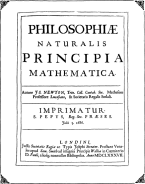• Newton therefore studied motion in detail, making many new observations.

Newton formulated his new observations (as well as those of others) in three fundamental Laws of Motion which govern all objects, including the planets.

These laws of motion were published in 1687 in Newton's monumental work Mathematical Principles of Natural Philosophy.

• When Newton talked about the motion of an object, he was referring to two general characteristics: speed and direction.

Together, these are also known as the linear momentum of the object.

An object at rest is simply a special case of motion, with zero speed.

#### 6.2 Newton's Laws of Motion

##### (Discovering the Universe, 5th ed., §2-5)
• Newton's First Law of Motion states:

 The motion of an object will remain unchanged unless a force acts on it.

In other words, an object will never change its speed or direction unless something comes along and forces it to do so.

This is an example of the principle of Conservation of Linear Momentum.

An object's resistance to changes in motion is known as inertia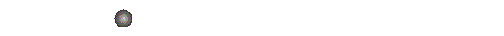• The First Law was actually stated a century earlier by Galileo (and even many centuries earlier than that by several others).

However, the First Law was in direct contradiction to the still-dominant teachings of Aristotle, who thought that all objects in motion will eventually come to rest of their own accord.

Newton therefore felt it necessary to make a forceful contradiction.

• Newton's Second Law of Motion describes how a force changes the motion of an object:

 F = ma

Here, F describes the force acting, m is the mass of the object, and a is its acceleration, the change in its motion.

• Like motion, force has both a value or "strength", and a direction in which it acts.

We commonly think of "acceleration" as a speeding up, but in physics it can also include a slowing down ("deceleration") as well as a change in direction.

• In the metric system, the unit of force is called a Newton, whose abbreviation is "N".

A Newton is equal to 1 kg•m/s2.

In the English system, the unit of force is the pound; it is equal to 4.45 N.

• We can deduce several things about moving objects by considering Newton's Second Law, F = ma:

• For example, the larger the force is, the larger the acceleration:• Second, the Second Law actually includes the First Law within it.

Question: why is this?

• Third, for an equal force, a larger mass must have a smaller acceleration, and vice versa:In other words, a larger mass has greater inertia than a smaller mass.

This is one way that we can measure an object's mass.

• Newton's Third Law of Motion describes two additional characteristics of forces:

 The force on an object is always due to another object, and that other object always feels an equal and opposite force.

You are familiar with this law from striking an object with your hand: the object moves as a result, but your hand also feels a force, and bounces back: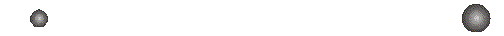Question: if the forces are equal, why does the smaller object bounce back faster?

#### 6.3 Forces in Nature

##### (Discovering the Universe, 5th ed., §2-5)
• Physicists have identified four fundamental forces in nature, all of which are consistent with Newton's Laws (or its subsequent generalizations):

• Gravity: an attractive force between objects with mass.

Gravity holds the Sun and planets together in the solar system, and holds stars together in galaxies.

You should be personally familiar with the effects of gravity, which holds you to the Earth and in general makes things "fall down".

We will discuss the gravitational force in more detail below.

• Electromagnetism: a force between objects with electric charge.

Electromagnetism holds atoms together, makes compasses point north, and is the source of starlight and auroras.

You should also be personally familiar with electromagnetism, via common devices such as electric appliances, refrigerator magnets, and the innumerable sources of light surrounding you.

We will discuss electromagnetism in more detail later on, in the contexts of electricity, magnetism, and light.

• Strong nuclear force: an attractive force between some subatomic particles.

The strong nuclear force holds atomic nuclei together, and we will talk about it more in that context.

The strong nuclear force is involved in the generation of energy in stars and in their explosive destruction known as type I supernovae.

Although this is probably not a force with which you have personal familiarity, it has been harnessed in nuclear power plants to provide electricity, and in various medical applications.

• Weak nuclear force: another force between some subatomic particles.

The weak nuclear force can change one type of subatomic particle into another in some situations such as radioactive decay, the generation of energy in stars, and in type II supernovae.

The weak nuclear force's other effects, observable in free particle collisions, are usually masked by the strong nuclear force, as its name implies.

The weak nuclear force shows up in the same sorts of technologies described above for the strong nuclear force.

We will consider the weak nuclear force in more detail in our discussion of radioactive decay.

• You are also personally familiar with contact forces, the result of electromagnetic forces acting within solids, liquids, and gasses:

•Normal Force: a repulsive force that opposes compression and prevents objects from passing through each other.

The normal force prevents planets and stars from collapsing down to zero size under the force of their own gravity (though not always!).

•Tension (Cohesion): an attractive force that holds objects together.

Cohesion prevents moons and planets from being torn apart by tidal forces (though not always!).

•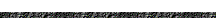Friction: an attractive force that opposes the sliding of objects past each other.

Friction between molecules in a gas falling into a black hole will cause it to heat up and emit radiation.

Question: if the force of gravity is pulling you downward, what force counteracts it to keep you in place while you are sitting or standing?

#### 6.4 The Universal Law of Gravity

##### (Discovering the Universe, 5th ed., §2-5)
• In order to complete his study of the motion of the planets, Newton had to combine his general Laws of Motion with a specific description of the force of gravity.

•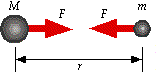Knowing the basic behavior of the planets from Kepler's Laws, Newton was able to determine an appropriate force law, the Universal Law of Gravitation: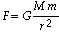Here, G is a constant, M and m are two masses, and r is the separation between them.

• Gravity is an attractive force, and in accordance with Newton's Third Law, the two masses feel equal and opposite forces.

• Gravity is relatively weak because of the small value of the gravitation constant G; in metric units,

G = 6.7 x 10-11 N•m2/kg2 .

Therefore, large masses are required to provide an appreciable force, e.g. the mass of the Earth is 6.0 x 1024 kg.

• Despite the Earth's large mass, the gravitational force holding you to the surface of the Earth, your weight, is still only a few hundred Newtons.

(Note: the distance r in the force law is the radius of the Earth, 6378 Km, as if all of its mass is concentrated at its center.)

#### 6.5 Gravity and Kepler's Laws

##### (Discovering the Universe, 5th ed., §2-6)
•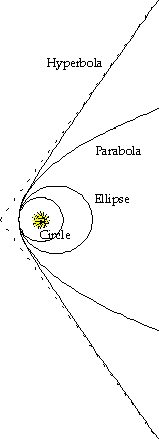By combining the Law of Gravitation with his Laws of Motion, Newton was able to mathematically derive all three of Kepler's Laws!

(In the process Newton also had to invent the mathematics of calculus!)

• Because the Sun is so much more massive than any of the planets, it has a very small gravitational acceleration, and can be taken as essentially motionless.

As described by Kepler, Newton found that all of the planets move around the Sun in circular and elliptical orbits, with the Sun located at a focus of each planet's orbit.

Objects with circular and elliptical orbits move around the Sun with a definite period, always between their perihelion and aphelion distances, and sweeping out equal areas in equal times.

• Newton also showed that Kepler's 3rd Law, relating semimajor axis and period, depends on the mass of whatever is being orbited: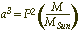As before, a is measured in AU and P is measured in years.

But now the mass M appears, and here is measured as multiples of the mass of the Sun.

This result provides a powerful tool for determining the mass of the Sun, as well any planet being orbited by a moon, simply by measuring a and P.

Question: Newton could immediately calculate the mass of which planets?

• Circular and elliptical orbits describe objects which are bound to the Sun.

Newton also demonstrated that there could be parabolic and hyperbolic orbits!

• A parabolic orbit describes an object which is marginally bound.

Such an object can escape from the Sun's gravity, but it will just barely reach an infinite distance.

Recall that a parabolic orbit has an eccentricity e = 1. Therefore, highly elliptical orbits (e > 0.9) approach a parabolic shape.

• An object in a hyperbolic orbit travels in a straight line until it nears the Sun and has its path deflected by gravity.

Such an object is not part of our solar system, but is instead simply passing through, and can be infinitely far from the Sun while still moving at high speed.

An object with a hyperbolic orbit is therefore said to be unbound.

A hyperbola has an eccentricity e > 1.

#### 6.6 Energy

##### (Discovering the Universe, 5th ed., §2-6)
• An important concept that arises from Newton's Laws of Motion is energy.

We all have an intuitive idea of what this means.

For example, when something moves very fast we say it "has a lot of energy".

We might also recognize that heat, electricity, and light have energy as well.

• Energy of motion is called kinetic energy.

Based on Newton's Laws, kinetic energy can be defined as: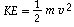This expression satisfies our intuition, which tells us that kinetic energy should depend not only on the object's speed v, but also on its mass m.

For example, a car travelling at 90 mph has much more energy than a baseball travelling at 90 mph.

• In the metric system, the unit of energy is the Joule, whose abbreviation is "J".

One Joule is equal to 1 kg·m2/s2, or equivalently 1 N·m.

A unit of energy you may be more familiar with is the food calorie, which is equal to 4184 J.

• You are probably more familiar with power, the rate of energy production or use (energy per unit time).

The metric unit of power is called a Watt, whose abbreviation is "W". One Watt is equal to 1 J/s.

Question: where have you seen the Watt appear in everyday usage?

#### 6.7 Potential Energy and Energy Conservation

##### (Discovering the Universe, 5th ed., §2-6)
•Consider an object which experiences a gravitational force.

If the object is thrown upward, it will have some initial speed but it will immediately begin to slow down and eventually stop, i.e. its kinetic energy decreases.

The object will then begin to move back downward, rapidly gaining speed as it is accelerated by gravity, and its kinetic energy increases.

• We intuitively think of an object with greater height as having, "potentially", more energy, because it will be moving faster when it reaches us.

We can therefore think of there being a potential energy associated with the object's height, which increases as it rises, and decreases as it falls, the opposite of kinetic energy.

• If we define the total energy E as the sum of the kinetic and potential energies,

E = KE + PE ,

then E will not change, i.e. it is conserved.

If kinetic energy increases, potential energy must decrease by an equal amount, and vice versa, so that E remains a constant value.

We can think of this as one kind of energy being converted into another.

• The potential energy described above is basically another way to characterize the force of gravity.

We can define potential energy for all of the fundamental forces, in such a way that energy is, in general, conserved.

As a result, energy conservation and conversion from one form to another are extremely important concepts in physics and astronomy, and we will see many applications, such as planet formation and solar thermal equilibrium.

#### 6.8 Gravitational Potential Energy

##### (Discovering the Universe, 5th ed., §2-6)
•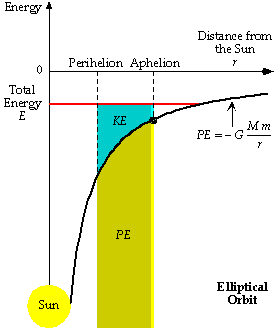The mathematical form of gravitational potential energy is very similar to the force law: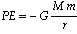Note that the potential energy is always a negative number, and reaches its maximum value of zero when the two masses are an infinite distance r apart.

• For planetary orbits, we can take the Sun to be fixed at position zero, and a planet to be a distance r away from it.

The potential energy of a planet in an elliptical orbit (e ~ 0.36) can then be plotted as shown in the picture at the right.

With each orbit around the Sun, the planet will move between its perihelion and its aphelion, and as it does so its energy will convert back and forth between potential and kinetic.

At perihelion, kinetic energy is a maximum and potential energy is a minimum; at aphelion, kinetic energy is a minimum and potential energy is a maximum.

Question: given that kinetic energy is closely related to speed, where was this characteristic of a planet's motion previously described?

Question: what can you say about the speed of a planet in a circular orbit?

•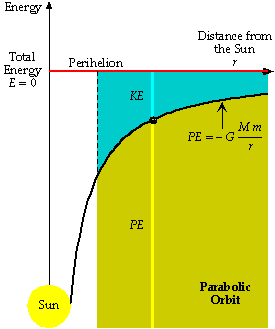Notice that, for the elliptical orbit above the total energy is negative (E < 0). This is true for all bound objects (those in circular or elliptical orbits).

• For marginally bound objects (those in parabolic orbits), the total energy is zero (E = 0).

Question: what is the speed of a marginally bound object which has moved an infinite distance from the Sun?

•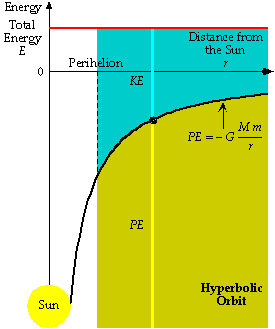For unbound objects (those in hyperbolic orbits), the total energy is positive (E > 0).

Question: what can you say about the speed of an unbound object at all times?

• You can further explore planetary motion and energy using this Java applet.

•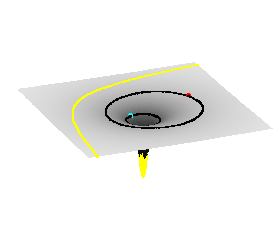We can get an intuitive feel for potential energy by "revolving" the potential energy curve into three dimensions.

Potential energy then forms a two-dimensional surface called a gravity well, with the Sun at the bottom and planetary orbits forming curves along it.

Imagine walking around the edge of a valley: as you walk higher you slow down, and as you descend you speed up; a planet does the same thing!

Question: in the image above you can see three types of orbits; can you tell what they are?

#### 6.9 Escape Velocity

##### (Discovering the Universe, 5th ed., Appendix)
• The escape velocity of an object such as a rocket is the speed required to depart from some point in a gravity well (such as the Earth's surface) and reach an infinite distance away.

This means that the object must be marginally bound or unbound, and so have at least zero total energy: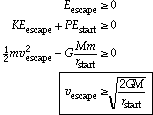• For the surface of the Earth, vescape = 11.2 Km/s ~ 40,000 Km/h.

For comparison, at the surface of the Sun, vescape = 618 Km/s, since its mass is 333,000 times that of the Earth!

• Newton knew about escape velocity, and used the idea in his Mathematical Principles to illustrate how gravity works; you can explore this yourself with this Java applet from the University of Virginia.

#### 6.10 Conservation of Angular Momentum

##### (Discovering the Universe, 5th ed., §2-5)
• Another important conservation law arises out of Newton's Laws of Motion, having to do with objects which rotate or revolve.

Much like linear momentum, objects have a tendency to keep rotating in the absence of external forces.

This "rotational inertia" is characterized by angular momentum, which can be loosely defined as:

AM ~ mr2/P

where m is the mass of the object, P is its period of rotation or revolution, and r is the distance from the center of rotation or revolution.

• The "direction" of angular momentum is also important; two rotating objects with the same mass and speed, but different axes of rotation, have different angular momenta.

• For many forces, including gravity, the angular momentum of a rotating or revolving object will be conserved, i.e. it will be constant.

Conservation of angular momentum is why the Earth always rotates once every 24 hours, and why its rotation axis remains (relatively) fixed in space as it orbits the Sun.

•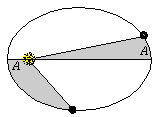Kepler's Second Law, which states that planets sweep out equal areas in equal times, is a consequence of conservation of angular momentum.

This can be seen from the above expression for angular momentum by recognizing that area A ~ r2 and time ~ P.

So, if AM ~ A/P is conserved, equal areas require equal times.

Question: where else have you seen conservation of angular momentum?

#### 6.11 Confirmations of Newton's Laws

##### (Discovering the Universe, 5th ed., §)
•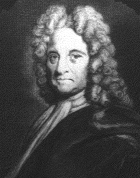A contemporary of Newton, Edmond Halley (1656-1742), had been studying comets, bright objects that appear briefly in the sky from time to time and move relative to the stars, like planets (though much faster).

Halley noticed that the comets of 1531, 1607, and 1682 had similar characteristics, and were separated by the same period of 76 years.

In 1684, Halley visited Newton in Cambridge, and told him about his suspicion that they were the same comet moving in a periodic orbit around the Sun.

It was only then that Halley learned of Newton's unpublished work on planetary motion.

Newton advised Halley that the comet's orbit must be elliptical, though much more elongated than a planet's.

• Halley applied Newton's laws to correctly predict the date and location of the comet's reappearance in 1758.

That comet is now known as Halley's Comet; its most recent passage was in 1986.

Halley's comet has an orbit with an eccentricity of 0.9673 and a semimajor axis of 17.9 AU (its aphelion of 35.3 AU takes it out beyond the planet Neptune!).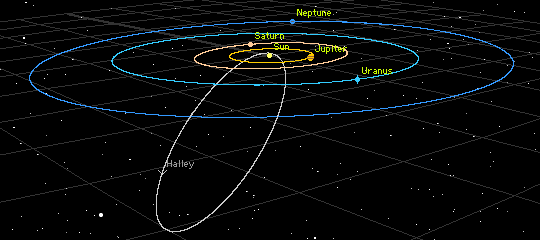• It wasn't long before astronomers had applied Newton's Laws to determine the masses of the known planets, by observing their interaction with their moons and with each other.

•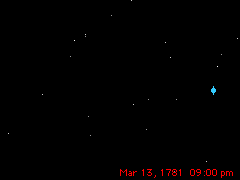In 1781, an amateur astronomer named William Herschel, who had built one of the most powerful telescopes of the time, noticed "a curious either nebulous star or perhaps a comet".

Subsequent observations revealed that this object moved relative to the stars, although more slowly than a comet.

Herschel soon realized that he had discovered a seventh planet, Uranus (and the first in recorded history!).

At its brightest, Uranus is barely visible to the naked eye in a dark sky.

Because of its slow motion, however, Uranus was always mistaken for a star by earlier observers.

•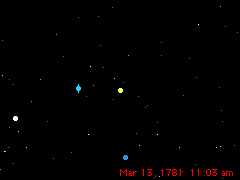Uranus has an orbital period of 84 years, but after 50 years it was clear that it wasn't following its predicted orbit.

Astronomers applied Newton's Laws to account for the gravitational pulls of Jupiter and Saturn, but that still did not remove all of the discrepancies.

John Couch Adams and Urbain-Jean-Joseph Le Verrier independently realized that a new planet beyond Uranus might cause these deviations.

In 1843 and 1845, respectively, they applied Newton's Laws to determine its position.

In 1846 Galle and d'Arrest at the Berlin Observatory followed up on Le Verrier's prediction, and quickly identified the eighth planet, Neptune.

Neptune moves even more slowly than Uranus, and it has an orbital period of 165 years.

Question: in the picture shown, looking down on the solar system, what effect would the gravity of the outer planet (Neptune) have on the speed of the inner planet (Uranus)?

• After some years, positional errors in Neptune's predicted orbit again suggested the presence of another large planet further out.

In 1905, another amateur astronomer, Percival Lowell, who had built his own observatory in Arizona, began a search for this planet, which he called Planet X.

After Lowell died in 1916, his successors at the Lowell Observatory continued the search.

Finally, in 1930, Clyde Tombaugh discovered Pluto, but its observation was fortuitous because its mass is so small that it doesn't have a significant effect on Neptune.

Better measurements of the outer planets' masses (by the Voyager spacecraft in 1970s and 1980s) have now removed the errors in Neptune's position.

•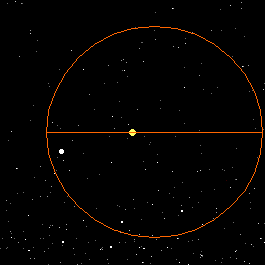Generally speaking, a planetary orbit should be fixed in space.

Question: what physical principle requires this?

However, another planet's gravitational pull can make a planet's orbit precess around the Sun.

Question: where have we already seen an example of a precessing orbit?

In 1845, Le Verrier discovered that Mercury's orbit also precesses around the Sun (note the changing orientation of the semimajor axis in the picture).

This is a very small effect, only 0.10 seconds of arc per orbit (43" per century), but even so it could not be explained by the other planets.

Le Verrier hypothesized that there might be another planet inside of Mercury's orbit, so close to the Sun that it was difficult to observe.

This planet, which Le Verrier called Vulcan, has never been found.

So, there was no good explanation for Mercury's precession using Newton's laws, but, like Neptune, the effect was small enough to attribute to measurement errors, and was largely ignored.

 The Halley's orbit, Uranus Motion, Uranus Passes Neptune, and Mercury's Precession animations, as well as the star chart background,were produced on a Macintosh with the Voyager II program, and are ©1988-93 Carina Software, 830 Williams St., San Leandro, CA 94577, (510) 352-7328. Used under license.   ©1996-2003 Scott R. AndersonLast update: 2003 April 10 Please send questions, comments, suggestions, or corrections to srca@mindspring.com. The material on this website may be reused as described under the Open Course License.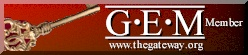The Gateway to Educational Materials (GEM) is the key to one-stop, any-stop access to thousands of high quality lesson plans, curriculum units and other education resources on the Internet! GEM is a project of the U.S. Department of Education. The Introduction to Astronomy Webbook is catalogued in the Gateway, and Scott R. Anderson is a member of the GEM Consortium.